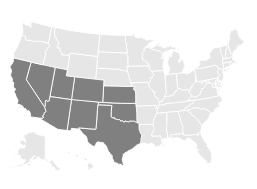Assignment: Euler and Hamiltonian Circuits

1. You and your friends want to tour the southwest by car. You will visit the nine states below, with the following rather odd rule: you must cross each border between neighboring states exactly once (so, for example, you must cross the Colorado-Utah border exactly once). Can you do it? If so, does it matter where you start your road trip? What fact about graph theory solves this problem?2. Below is a graph representing friendships between a group of students (each vertex is a student and each edge is a friendship). Is it possible for the students to sit around a round table in such a way that every student sits between two friends? What does this question have to do with paths?